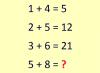BRAIN TEASERSBrain Teasers User Profile

 Calculate 5+8 If 1+4=5, 2+5=12 and 3+6=21 then 5+8=?